# Math Olympiad Test: Statistics- 4 - Class 10

Test Description

## 10 Questions MCQ Test Olympiad Preparation for Class 10 - Math Olympiad Test: Statistics- 4

Math Olympiad Test: Statistics- 4 for Class 10 2023 is part of Olympiad Preparation for Class 10 preparation. The Math Olympiad Test: Statistics- 4 questions and answers have been prepared according to the Class 10 exam syllabus.The Math Olympiad Test: Statistics- 4 MCQs are made for Class 10 2023 Exam. Find important definitions, questions, notes, meanings, examples, exercises, MCQs and online tests for Math Olympiad Test: Statistics- 4 below.
 1 Crore+ students have signed up on EduRev. Have you?
Math Olympiad Test: Statistics- 4 - Question 1

### The mean of six numbers is 21. If one number is excluded, then their mean is 19, the excluded number is ______.

Detailed Solution for Math Olympiad Test: Statistics- 4 - Question 1

Let the excluded number be x and the sum of rest of numbers be y.

Then,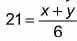⇒ 126 = x + y

Also, 19 = y/5

⇒ y = 95

∴ From (i), x = 31.

Math Olympiad Test: Statistics- 4 - Question 2

### If 7, 2, 9 and 5 occur with frequencies 2, 3, 6 and 4 respectively,  then the arithmetic mean is _____.

Detailed Solution for Math Olympiad Test: Statistics- 4 - Question 2

Arithmetic mean =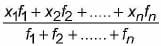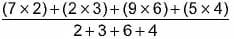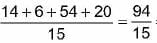= 6.27

Math Olympiad Test: Statistics- 4 - Question 3

### The mean of 20 observations is 12.5. By error, one observation was noted as –15 instead of 15. Then the correct mean is _____.

Detailed Solution for Math Olympiad Test: Statistics- 4 - Question 3

The incorrect sum of observations = 12.5 x 20 = 250

Now, correct sum = 250 - (-15) + 15 = 280

∴ Correct mean = 280/20 = 14

Math Olympiad Test: Statistics- 4 - Question 4

Find the value of p , if the mean of the following data is 20.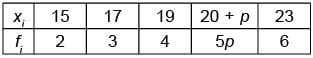Detailed Solution for Math Olympiad Test: Statistics- 4 - Question 4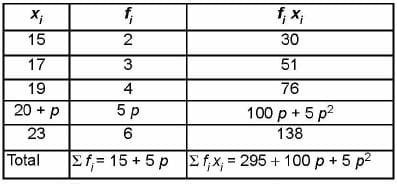Mean =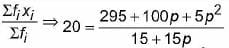⇒  20 (15 + 5 p) = 295 + 100 p + 5 p2

⇒  300 + 100 p = 295 + 100 p + 5 p2

⇒ 5p2 = 5

⇒ p2 = ±1 ⇒ p = 1 ( ∵p ≠ - 1)

Math Olympiad Test: Statistics- 4 - Question 5

IfDetailed Solution for Math Olympiad Test: Statistics- 4 - Question 5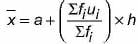⇒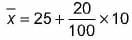= 25 + 2 = 27

Math Olympiad Test: Statistics- 4 - Question 6

A bus maintains an average speed of 60 km/hr while going from P to Q and maintains an average speed of 90 km/hr while coming back from Q to P. The average speed of the bus is _____.

Detailed Solution for Math Olympiad Test: Statistics- 4 - Question 6

Average speed = Total distance covered/Total time taken

Let x be the distance between P and Q.

Thus, time taken by bus from P to Q (t1) = x/60

Thus, time taken by bus from Q to P (t2) = x/90

Total distance covered = 2x  and total time taken = t1 + t2

Average speed,  =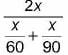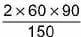= 72 km/hr

Math Olympiad Test: Statistics- 4 - Question 7

Candidates of four school appear in a mathematics test. The data is as follows: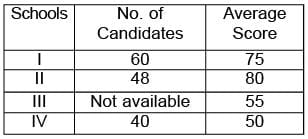If the average score of the candidates of all the four schools is 66, find the number of candidates that appeared from school III.

Detailed Solution for Math Olympiad Test: Statistics- 4 - Question 7

Let the number of candidates that appeared from school III- be x.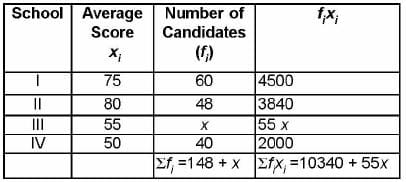Average score of the candidates of all the four schools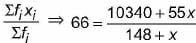⇒ 9768 + 66 x = 10340 + 55 x

⇒ 11x = 572

⇒ x = 52

Math Olympiad Test: Statistics- 4 - Question 8

Fill in the blanks.
(i) For a given data with 110 observations the ‘less than ogive’ and ‘more than ogive’ intersect at (18, 20). The median of the data is __P__.
(ii) The curve is drawn by taking upper limit of class interval along x-axis and cumulating frequency along y-axis is __Q__ than ogive.
(iii) The mean of 50 number s is 18, the new mean will be __R__ if each observation is increased by 4.
(iv) The mean of seven consecutive natural numbers is 20, then the largest number is __S__ and smallest number of them is __T__.
P, Q, R, S, T

Detailed Solution for Math Olympiad Test: Statistics- 4 - Question 8

(i) The intersection point of less than ogive and more than ogive, the x-coordinate is the median. Median is 18.

(ii) Less than ogive is drawn by taking the upper limit of class interval along x-axis and their corresponding less than cumulative frequencies along y-axis.

(iii) Sum of 50 numbers = 50 x 18 = 900

If each number is increased by 4, then

new sum = 900 + 4 x 50 = 1100

∴ New Mean = 1100/50 = 22

(iv) Let seven consecutive numbers are x, x + 1, x + 2, ...., x + 6.

Mean =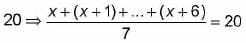⇒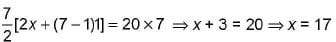Smallest number x = 17 Largest number = x + 6 = 17 + 6 = 23.

Math Olympiad Test: Statistics- 4 - Question 9

Find the mean, median and mode of the following data.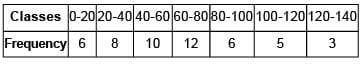Mean, Median, Mode

Detailed Solution for Math Olympiad Test: Statistics- 4 - Question 9

To find the mean let us put the data in the table given below:Thus, the mean of the given data is 62.4.
Now, to find the median let us put the data in the table given below:Now, N = 50 ⇒ N/2 = 25.
The cumulative frequency just greater than 25 is 36 and the corresponding class is 60 – 80.
Thus, the median class is 60 – 80.
∴ l = 60, h = 20, N = 50, f = 12 and cf = 24.
Now,Hence, Mean = 62.4, Median = 61.67 and Mode = 60.21

Math Olympiad Test: Statistics- 4 - Question 10

Find the median for the following distribution of workers.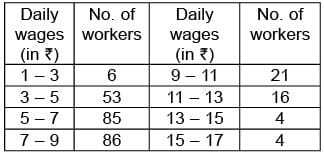Detailed Solution for Math Olympiad Test: Statistics- 4 - Question 10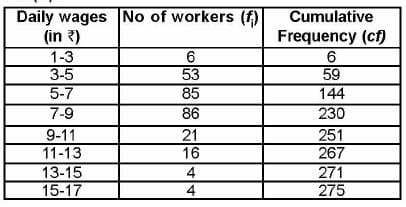Here,n = 275 ⇒ n/2 = 137.5

Median class is 5 - 7

Median =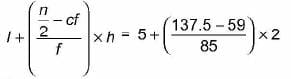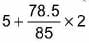= 5 + 1.84 = 6.84

## Olympiad Preparation for Class 10

11 videos|36 docs|201 tests